Courses

#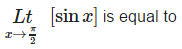a)1b)– 1c)0d)none of theseCorrect answer is option 'C'. Can you explain this answer? Related Test: Test: Continuity And Differentiability (CBSE Level) - 1

## JEE Question

By Mansi Sharma · Mar 12, 2020 ·JEE
Vikas Saini answered Mar 12, 2020
Santosh sahoo discovers sin90* as zero LOL.sin90* is not perfact 1 in limits,it is slightly less than 1,so it is zero in GIF and LHL and RHL has cos

Shashmith Shashmith answered Mar 24, 2020

Rathod Guru answered 3 weeks ago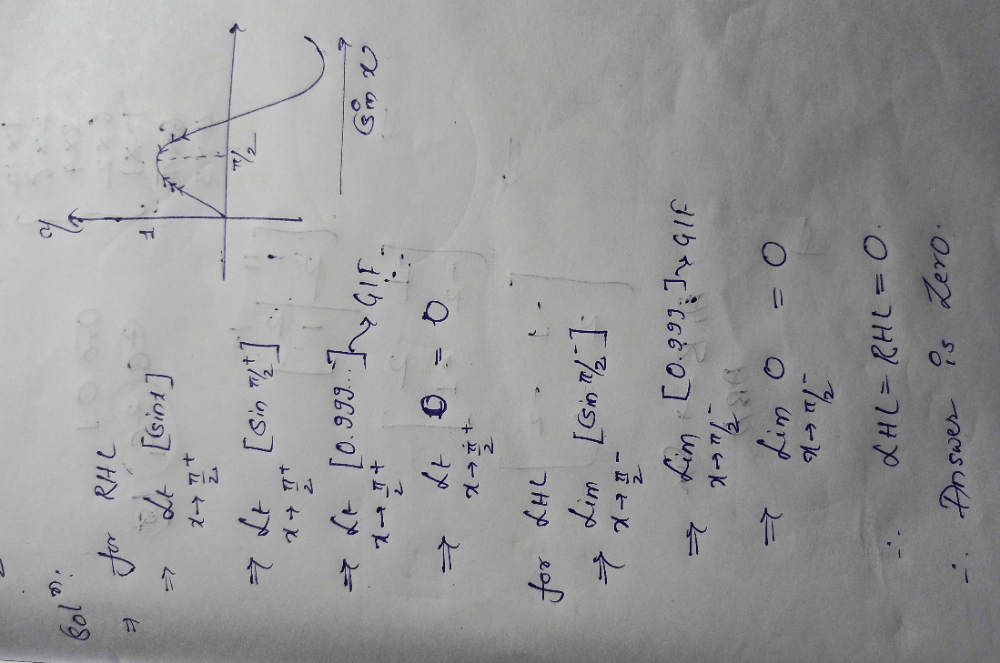Vishwajeet Dhumal answered Apr 08, 2020
We have to find the the greatest integer part of the value [sinx]. we know that sin pi/2 = 1 but since the limit is approaching pi/2 but is not completely pi/2, therefore we can conclude by the rules of greatest integer function that the value of function greater than 0 and slightly less than 1 is always zero(only if it is the greatest integer function).

Rabin Mondal answered Apr 15, 2020
0

Silent Nisarg answered Apr 14, 2020

Khaja Moinuddin answered 4 weeks ago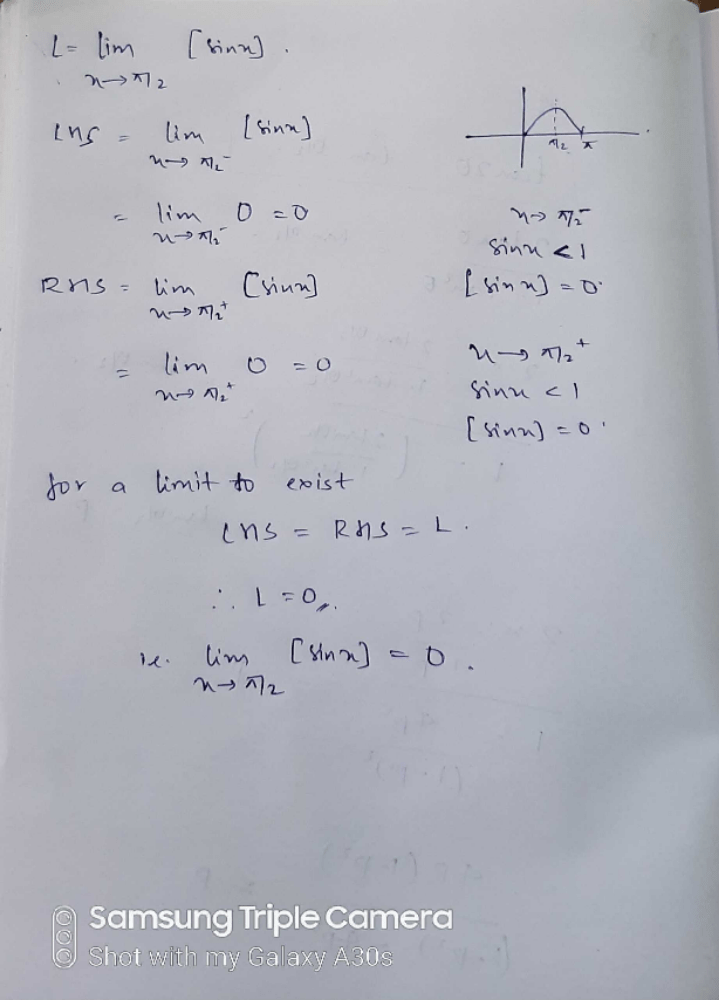Vikas Saini answered 2 weeks ago
C is correct

Rajendra Singh Bhandari answered 2 weeks ago
By putting pie/2 in box of sinx it will be less than 1 so box value will be zero

Mahesh Botsa answered 3 days ago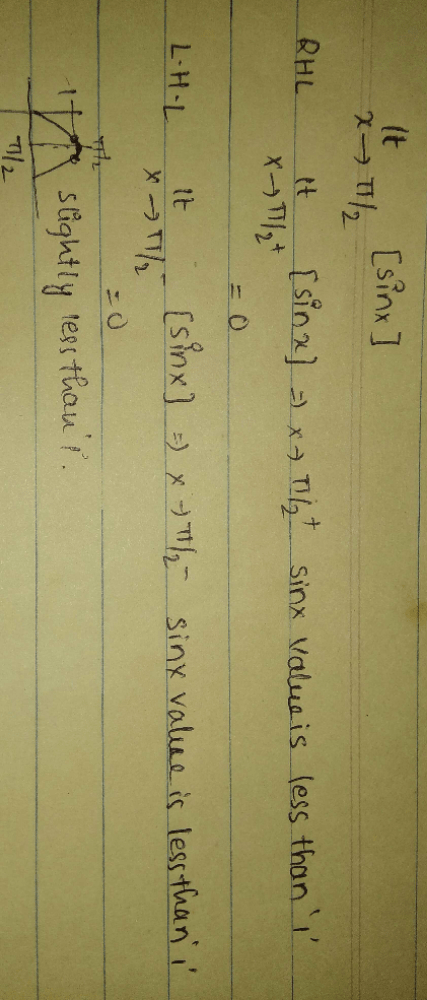Harsh Gujalwar answered 2 days ago
The ans must be zero as theta tends to pie hence sin theta tend to 1 but never equal to 1 hence gif is 0

Harun Shankar answered 2 weeks ago
At exactly x=1 without limits step of sinx is 1 but limit x tends to means it approaches to not equal to
then the value is always less than 1 if we apply step function it is zero

Suresh Kumar answered 3 weeks ago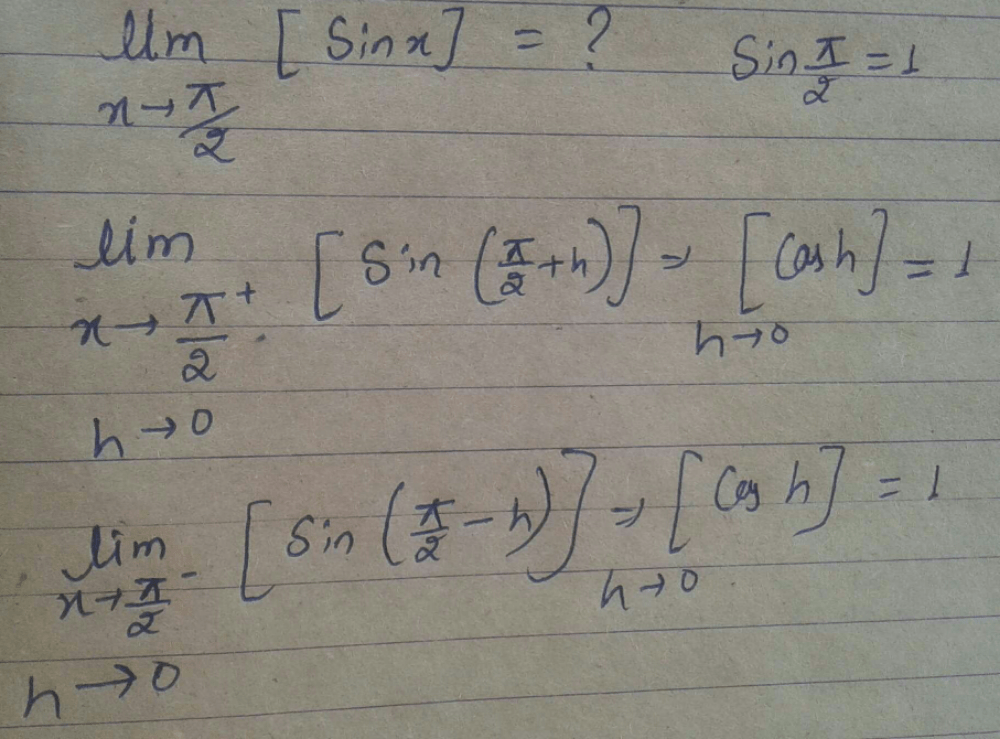Sagar Naik answered Apr 17, 2020
Bez it is in greatest integer function.....[sinx]

Yes c is correct

Shweta Singh answered 3 weeks ago
Π/2=90• which is equal to 0

Nisha Sharma answered 1 week ago

Aishwary Omar answered Apr 21, 2020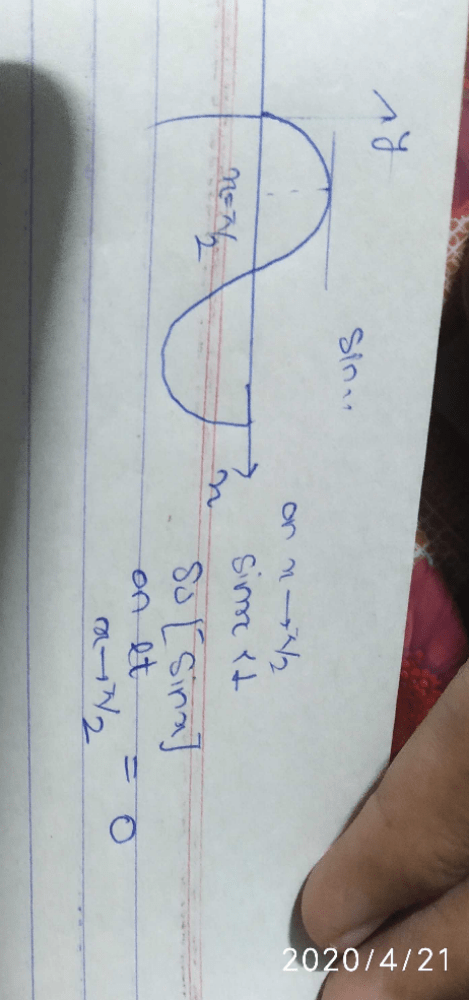Rekha Gupta answered 3 weeks ago
1

Shubham Choudhary answered 4 weeks ago
With the observation of graph of Sin X we can see that
limit X tends to pie/2+ and X tends to pie/2- is less than one so it's gif is zero

Santosh Sahoo answered Apr 01, 2019
Sin pi by 2 = sin90degree = 0
so the option c is correct......l hope you understand

Armesha Sharma answered 4 days ago
Why do u mean by cos???

Bhukya Nithin answered 2 weeks ago
When we consider the value of sin[pi/2] it gets in between 0 and 1.
And we know that, [ ] this bracket indicates the greatest integer function.
therefore,when we consider the both left hand limit and right hand limit they both equal each other to 0.
that's why 0 is the correct answer.

Chethana R Veena answered Apr 19, 2020
C

Sin(π\2)=1 so, option A is correct answer

Mahalakshmi Maha answered Apr 25, 2020
Actually sinπ/2 is 1, but the question is greatest integer function of sinx i.e, [sinx] and the value is not exactly 1 as limit approaches π/2 and not exactly π/2. So it is to be considered as 0 by the greatest integer function rule. So answer is 0. Option C is correct.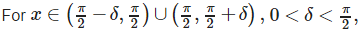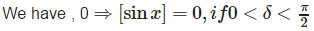Shan Mukherjee answered Apr 12, 2020
Sin(x) tending to 90° means either greater than 90° or less then 90° in both the cases it will less the zero according to it's graph
hence gif of any number less the one is absolute zero.
good question

C Ramamohanreddy answered Apr 21, 2020
The question really mean that if x approach 90*then sinx approach what, yes it approach 1,but not exactly 1,it may be 0.99 something ,you may think it may be 1.01 but sin value doesn't exceed 1.so G.I.F of less than one is zero

Sontosh Dey answered Apr 15, 2020
Yes option c is correct

Ganji Manaswini answered Apr 21, 2020

Raghav Shukla answered 3 weeks ago
Just see limit is in greatest integer function , since range of sinx is -1 to 1
.From graph you can see when you see left hand limit you got a number less than 1 whose greatest integer is zero(0) similarly when you see right hand limit you got a number less than 1 whose greatest integer is zero(0)
SINCE RHL=LHL=0
SO LIMIT OF FUNCTION EXISTS AT pi/2 and equal to zero(0)

Nandlal Rajak answered Apr 05, 2020
Sin90=1

Rocky Srk answered Mar 29, 2020
Sinx has value a little less then 1 in limit... here sinx is under integral function [ ] so option C is correct...

Ankit Singh answered Apr 14, 2020
Option c is the correct answer

Prashant Kumar answered 4 weeks ago
A

Akhils Pillai answered 4 weeks ago
Sinpi/2=0

Konduru Premsai answered Apr 28, 2020
D) As RHL not equal to LHL.

Shubham Nayan answered 2 weeks ago
In these types of questions we have to consider g.i.f .As sinx is approaching 1 i.e it is less than 1, the answer will be 0.But if the whole limit would have been in the gif the answer would be 1.

Answer is C BECAUSE SINX IS LESS THAN 1 AND BOX OR GREATEST INTEGER FUNCTION OF LESS THAN 1 IS ZERO

Stone Smith answered Mar 28, 2020
Op 1

Ravi Teja answered Mar 30, 2020
As [ ]is not given as g.i.f ans may be opt A
even if [ ]is given as g.i.f then limit does not exists....

Vijendra Vaishya answered 4 weeks ago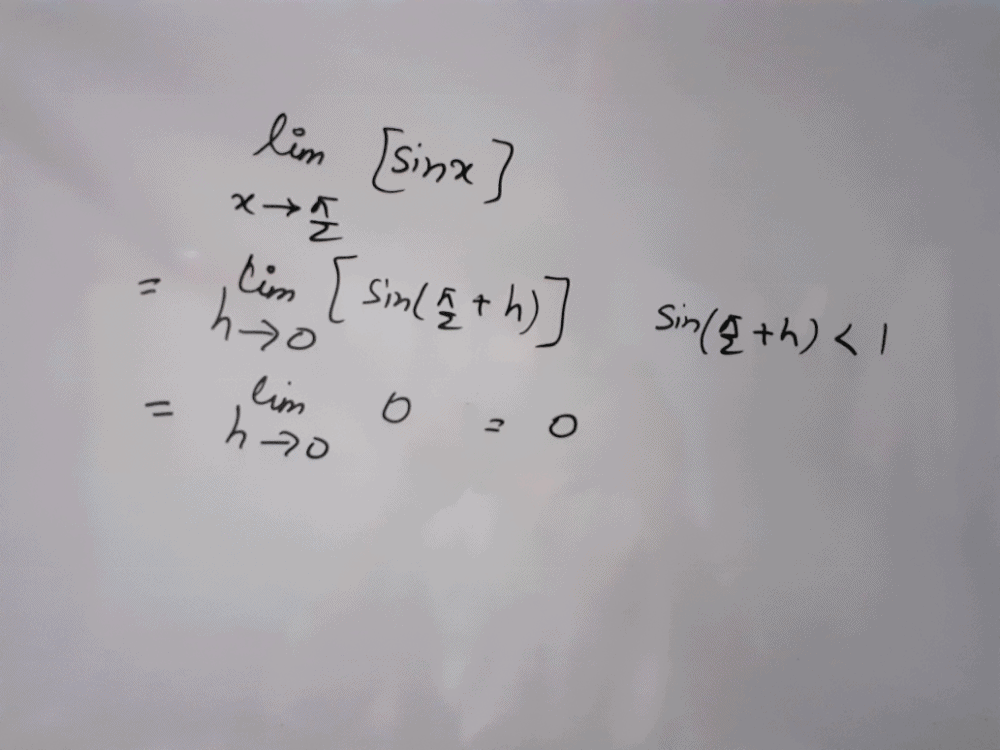Since it is approaching towards pi/2 not exact pi/2 so the value is not exact 1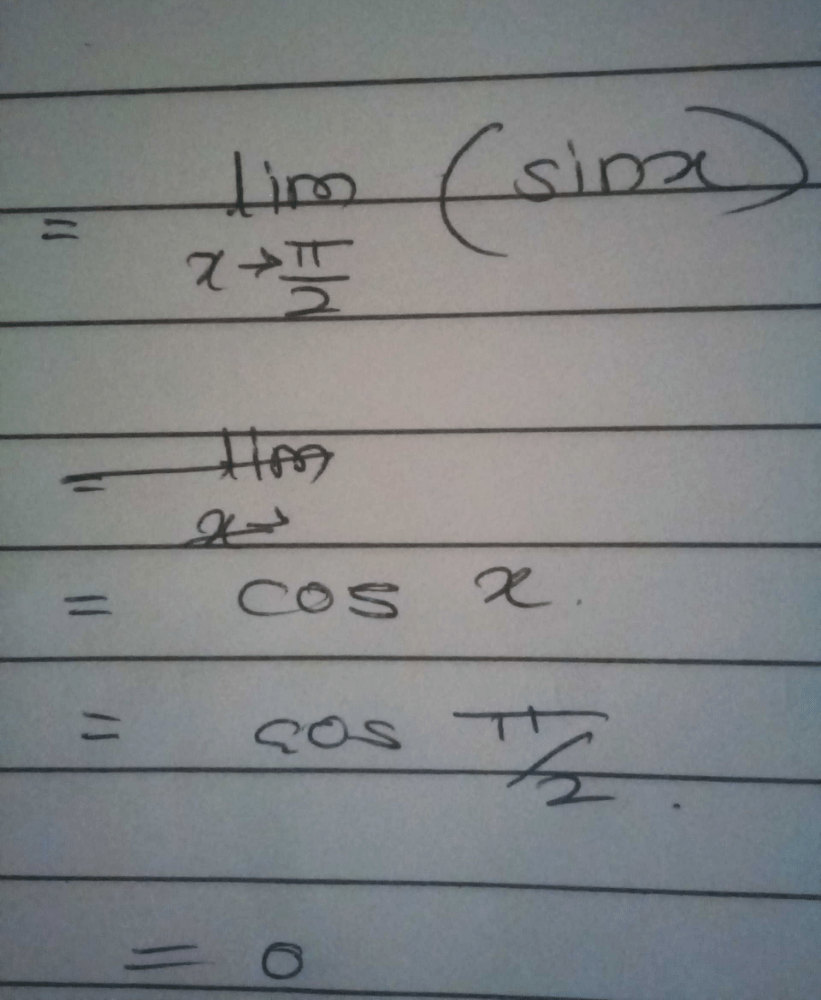Its Kings Club answered 4 days ago
It is not an indeterminate form so limit will be applied directly.
sin90 is 0